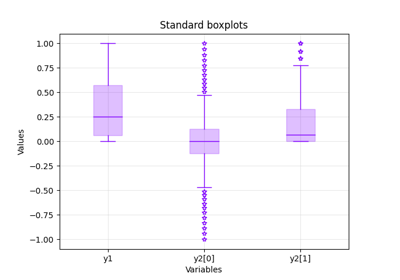gemseo / post / dataset

# boxplot module¶

Draw the boxplots of some variables from a Dataset.

A boxplot represents the median and the first and third quartiles of numerical data. The variability outside the inter quartile domain can be represented with lines, called whiskers. The numerical data that are significantly different are called outliers and can be plotted as individual points beyond the whiskers.

Bases: DatasetPlot

Draw the boxplots of some variables from a Dataset.

Parameters:
• dataset (Dataset) – The dataset containing the data to plot.

• *datasets (Dataset) – Datasets containing other series of data to plot.

• variables (Sequence[str] | None) – The names of the variables to plot. If None, use all the variables.

• center (bool) –

Whether to center the variables so that they have a zero mean.

By default it is set to False.

• scale (bool) –

Whether to scale the variables so that they have a unit variance.

By default it is set to False.

• use_vertical_bars (bool) –

Whether to use vertical bars.

By default it is set to True.

Whether to add the confidence interval (CI) around the median; a CI is also called notch.

By default it is set to False.

By default it is set to True.

• **boxplot_options (Any) – The options of the wrapped boxplot function.

Raises:

ValueError – If the dataset is empty.

execute(save=True, show=False, file_path=None, directory_path=None, file_name=None, file_format=None, properties=None, fig=None, axes=None, **plot_options)

Execute the post-processing.

Parameters:
• save (bool) –

If True, save the plot.

By default it is set to True.

• show (bool) –

If True, display the plot.

By default it is set to False.

• file_path (str | Path | None) – The path of the file to save the figures. If None, create a file path from directory_path, file_name and file_format.

• directory_path (str | Path | None) – The path of the directory to save the figures. If None, use the current working directory.

• file_name (str | None) – The name of the file to save the figures. If None, use a default one generated by the post-processing.

• file_format (str | None) – A file format, e.g. ‘png’, ‘pdf’, ‘svg’, … If None, use a default file extension.

• properties (Mapping[str, DatasetPlotPropertyType] | None) – The general properties of a DatasetPlot.

• fig (None | Figure) – The figure to plot the data. If None, create a new one.

• axes (None | Axes) – The axes to plot the data. If None, create new ones.

• **plot_options – The options of the current class inheriting from DatasetPlot.

Returns:

The figures.

Raises:

AttributeError – When the name of a property is not the name of an attribute.

Return type:

list[Figure]

color: str | list[str]

The color(s) for the series.

If empty, use a default one.

colormap: str

The color map.

dataset: Dataset

The dataset to be plotted.

fig_size: tuple[float, float]

The figure size.

property fig_size_x: float

The x-component of figure size.

property fig_size_y: float

The y-component of figure size.

font_size: int

The font size.

property labels: Mapping[str, str]

The labels of the variables.

legend_location: str

The location of the legend.

linestyle: str | list[str]

The line style(s) for the series.

If empty, use a default one.

marker: str | list[str]

The marker(s) for the series.

If empty, use a default one.

opacity_level: ClassVar[float] = 0.25

The opacity level for the faces, between 0 and 1.

property output_files: list[str]

The paths to the output files.

title: str

The title of the plot.

xlabel: str

The label for the x-axis.

xmax: float | None

The maximum value on the x-axis.”.

If None, compute it from data.

xmin: float | None

The minimum value on the x-axis.

If None, compute it from data.

ylabel: str

The label for the y-axis.

ymax: float | None

The maximum value on the y-axis.

If None, compute it from data.

ymin: float | None

The minimum value on the y-axis.

If None, compute it from data.

zlabel: str

The label for the z-axis.

zmax: float | None

The maximum value on the z-axis.

If None, compute it from data.

zmin: float | None

The minimum value on the z-axis.

If None, compute it from data.

## Examples using Boxplot¶Boxplot

Boxplot